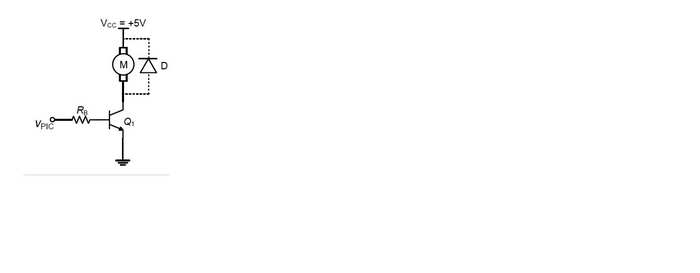# BJT Transistor w/ motor

• Engineering
• phsyics_197

## Homework Statement

You want to use a PIC microcontroller to drive a motor in a clockwise direction at either 0% speed (when the PIC output is 0V) or full speed (when the PIC output is +5V). This motor draws 500mA at +5V while the output of the used PIC is limited to +5V and 20mA. To solve this problem you decided to use a BJT transistor that withstands 500mA as an interface between the PIC and the motor as shown in Fig 2. This transistor will play the role of a switch that turns on and off the motor while it is also able to handle the required current by the motor.

Notice that there is no need to add a limiting resistor RC in series with Q1 collector because the motor internal resistance will limit the collector current IC to 500mA at VCC = +5 V and VCE(Sat) = 0.21V.

The selected transistor has the following specifications extracted from its datasheet:
- VBE = 0.7V when the EBJ is forward biased
- 300 < hFE = Beta < 400, means that you can obtain a current gain of at least 300 (for Beta minimal) at the edge of saturation (EOS).
- max IC (Sat) = 4000mA, meaning that this transistor can withstand max 4000mA.
- VCE(Sat) = 0.21V an estimate of VCE at saturation
- VCEO = 40V, means that this transistor can handle VCE voltages up to 40V when it is
turned off
(a) Select a standard value RB that guarantees the operation of your transistor in saturation.lots.

## The Attempt at a Solution

I am not 100% sure, because there are a lot of numbers being thrown at me, of what to do.

How I tried it was: When PIC = +5V, the current can not be larger than 20mA.

5 - VBE / R = Ib = 4.3/R < 20mA

Imotor = 500mA = Ic
Ic = (Beta)Ib

I plugged in random Resistor values to obtained an Ib less than 20mA and Beta to be between 300 and 400. I got 3.3kOhm (there are a whole range of them.

What I need to know now (if that was correct) how to prove everything else to ensure 3.3kOhm was correct.